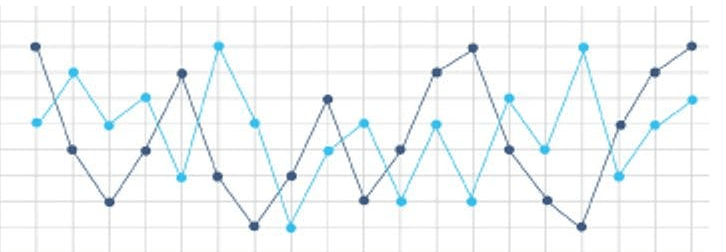Net-informations.com

# Python Statistical Data Analysis

Python is a general-purpose language that's powerful, easy to learn and fast to code. It's also useful in the development of complex numeric and scientific applications . Python grows rapidly becoming the programming language of choice for data-scientists .Statistical modelling gives you the ability to asses, understand and make predictions about the data, it is at the very bottom of inferential statistics and can be considered of those "must know" topics. Python's statistical libraries,such as NumPy, Pandas, and Matplotlib etc. , are comprehensive, popular, and widely used data analytical tools that will help you in working with huge amount of data. The following chapters will introduce the use of Python for statistical data analysis , using data stored as Pandas DataFrame objects.

net-informations.com (C) 2022    Founded by raps mk